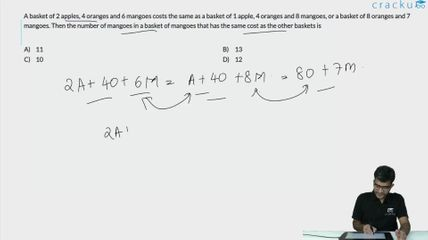Question 53

# A basket of 2 apples, 4 oranges and 6 mangoes costs the same as a basket of 1 apple, 4 oranges and 8 mangoes, or a basket of 8 oranges and 7 mangoes. Then the number of mangoes in a basket of mangoes that has the same cost as the other baskets is

Solution

Let the cost of an apple, an orange and a mango be a, o, and m respectively.

Then it is given that:

2a+4o+6m = a+4o+8m

or a = 2m.

Also, a+4o+8m = 8o + 7m

10m-7m = 4o

3m = 4o.

We can now express the cost of a basket in terms of mangoes only:

2a+4o+6m = 4m+3m+6m = 13m.

### View Video Solution